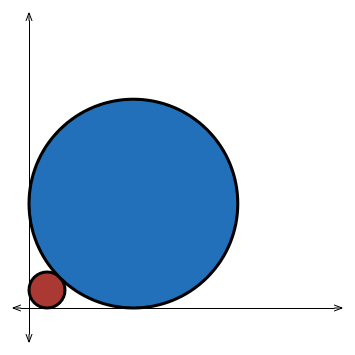# JEE Circles 1

Geometry Level 3$C_1$ is a circle of radius 1 unit touching the x-axis and the y-axis. $C_2$ is another circle with radius $R>1$ and touching the axes and $C_1$. Then calculate $\lfloor 1000R\rfloor$

×